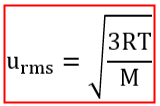# Problem: Mars has an atmosphere composed almost entirely of carbon dioxide, with an average temperature of -63°C.What is the rms speed of a molecule in Mars' atmosphere? Assume  R = 8.315 J/mol•K.Express your answer to two significant figures and include the appropriate units.

###### FREE Expert Solution

The root mean square (rms) speed predicts the speed of gaseous molecules with a certain kinetic energy at a specific temperatureWhere: R is the universal gas constant (8.315 J/mol-K)

T is the temperature in Kelvin

M is the molar mass of the gas (in kg/mol)

We are asked to determine the urms of carbon dioxide in Mars where the temperature is -63°C.

83% (430 ratings)###### Problem Details

Mars has an atmosphere composed almost entirely of carbon dioxide, with an average temperature of -63°C.

What is the rms speed of a molecule in Mars' atmosphere? Assume  R = 8.315 J/mol•K.

Express your answer to two significant figures and include the appropriate units.